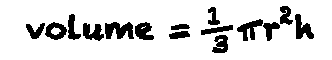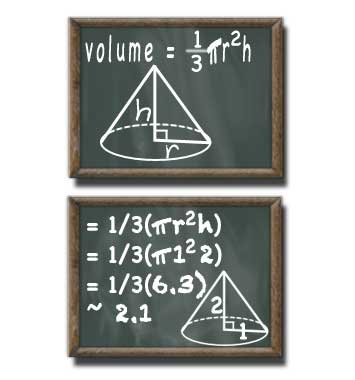# Volume of a Cone Calculator

## Cone Volume

#### For Finding Volume of a Cone with Circular Base

Result:
2.094height (h) decimals

A cone with a base radius of 1 units and a height of 2 units has a volume of 2.094 cubed units.

This calculator and more easy to use calculators waiting at www.KylesCalculators.com### Calculating the Volume of a Cone:

If a cone has a flat bottom, meaning the height and radius meet at right angles, then this formula can be used to find of volume ('V') of that cone (also know as a right circular cone):

V = 1/3(PI*r2h)

In plain english the volume of a cone can be calculated by taking one-third of the result of the radius squared, multiplied by the height, multiplied by the mathematical constant pi.

Here is a step-by-step case that illustrates how to find to volume of a cone with a radius of 2 feet and a height of 3 feet. Note that in order to save space (and because pi cannot be determine precisely) a limited number of decimal places are used, the symbol ~ denotes that this answer is an approximation.

• V = 1/3(pi * r2 * h)
• = 1/3(pi * 22 * 3)
• = 1/3(pi * 12)
• = 1/3(37.7)
• ~ 12.6 cubic feet